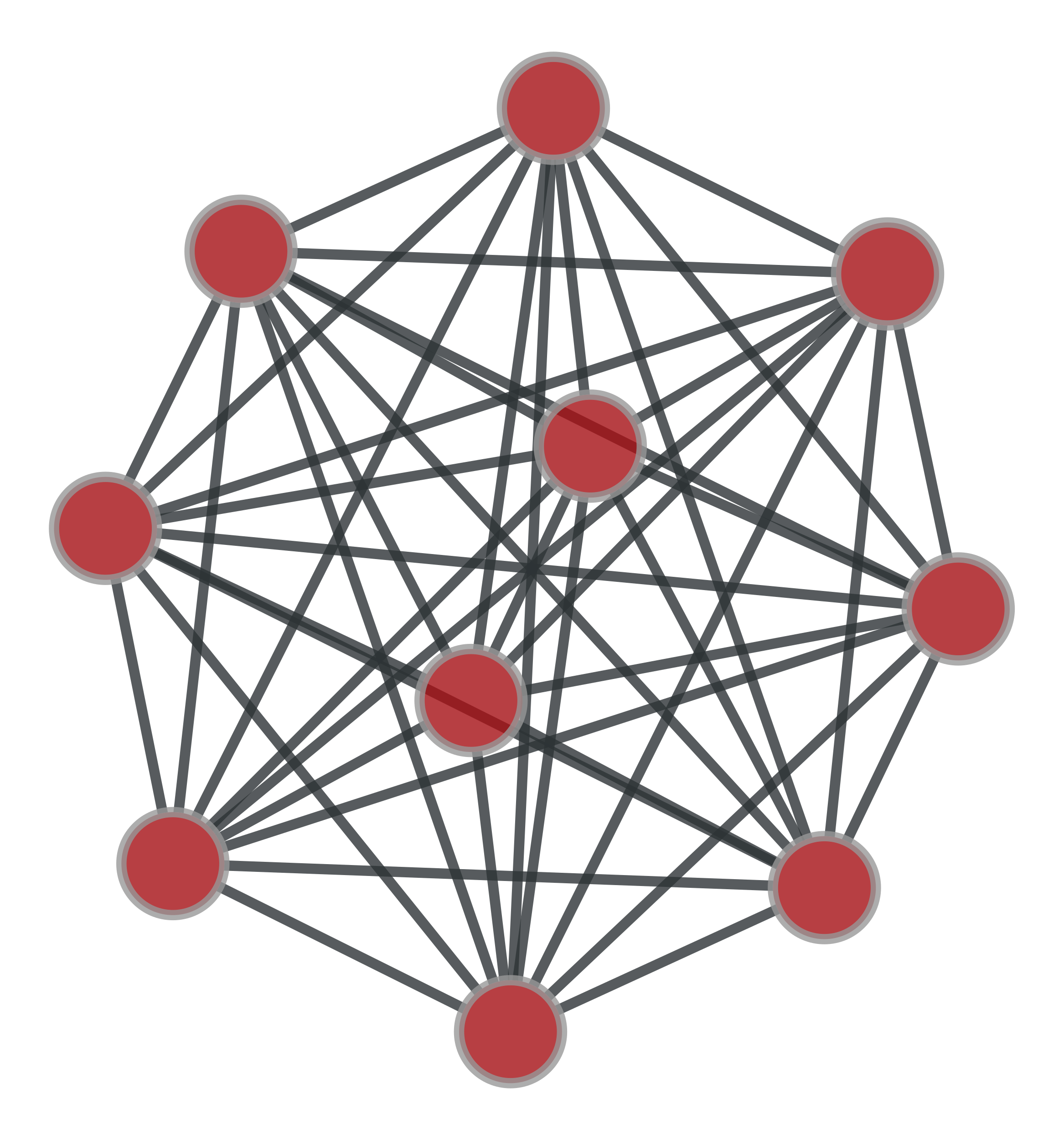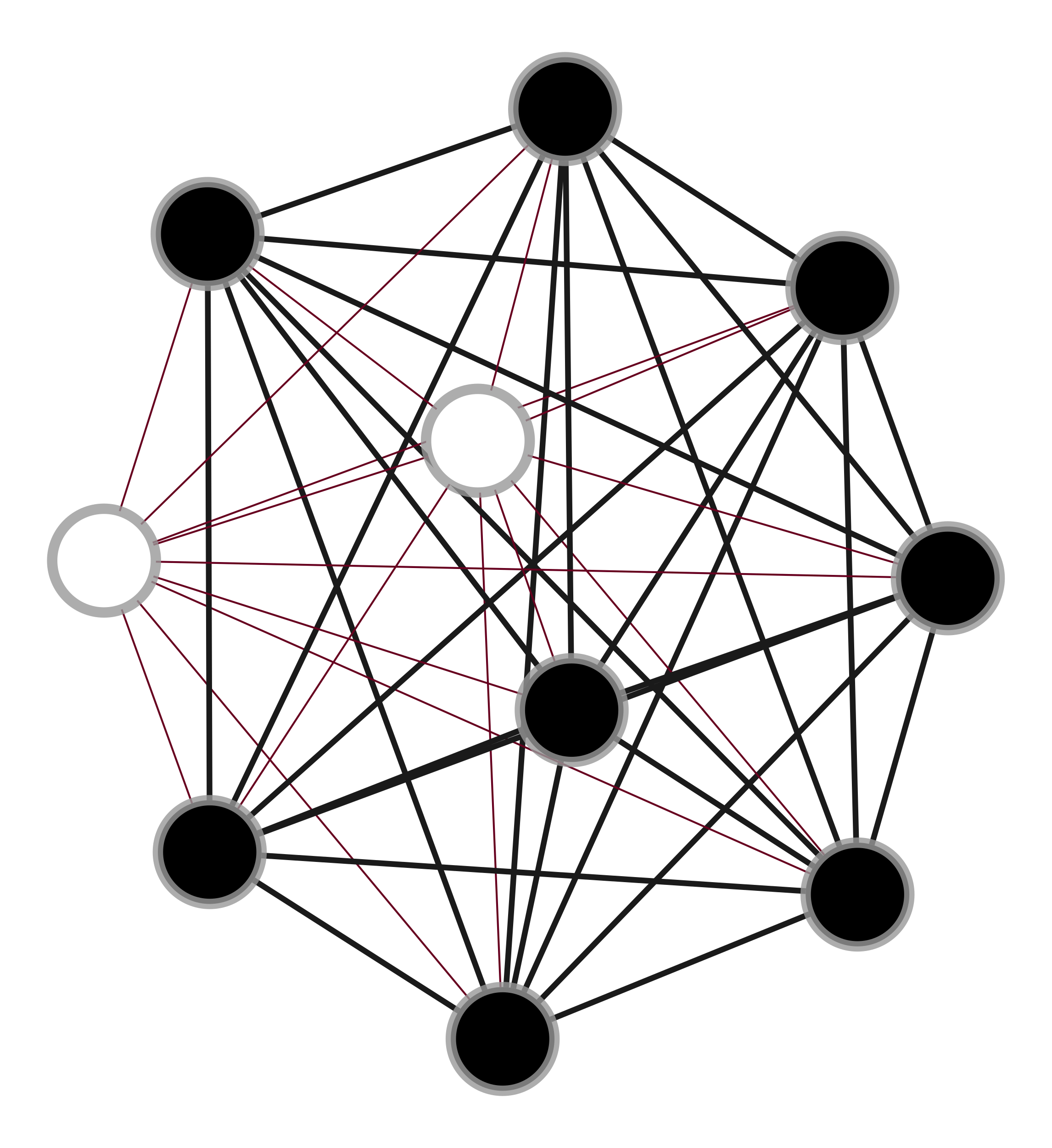# graph_tool.topology.subgraph_isomorphism#

graph_tool.topology.subgraph_isomorphism(sub, g, max_n=0, vertex_label=None, edge_label=None, induced=False, subgraph=True, generator=False)[source]#

Obtain all subgraph isomorphisms of sub in g (or at most max_n subgraphs, if max_n > 0).

Parameters:
subGraph

Subgraph for which to be searched.

gGraph

Graph in which the search is performed.

max_nint (optional, default: 0)

Maximum number of matches to find. If max_n == 0, all matches are found.

vertex_labelpair of VertexPropertyMap (optional, default: None)

If provided, this should be a pair of VertexPropertyMap objects, belonging to sub and g (in this order), which specify vertex labels which should match, in addition to the topological isomorphism.

edge_labelpair of EdgePropertyMap (optional, default: None)

If provided, this should be a pair of EdgePropertyMap objects, belonging to sub and g (in this order), which specify edge labels which should match, in addition to the topological isomorphism.

inducedbool (optional, default: False)

If True, only node-induced subgraphs are found.

subgraphbool (optional, default: True)

If False, all non-subgraph isomorphisms between sub and g are found.

generatorbool (optional, default: False)

If True, a generator will be returned, instead of a list. This is useful if the number of isomorphisms is too large to store in memory. If generator == True, the option max_n is ignored.

Returns:
vertex_mapslist (or generator) of VertexPropertyMap objects

List (or generator) containing vertex property map objects which indicate different isomorphism mappings. The property maps vertices in sub to the corresponding vertex index in g.

Notes

The implementation is based on the VF2 algorithm, introduced by Cordella et al. [cordella-improved-2001] [cordella-subgraph-2004]. The spatial complexity is of order $$O(V)$$, where $$V$$ is the (maximum) number of vertices of the two graphs. Time complexity is $$O(V^2)$$ in the best case and $$O(V!\times V)$$ in the worst case [boost-subgraph-iso].

References

L. P. Cordella, P. Foggia, C. Sansone, and M. Vento, “An improved algorithm for matching large graphs.”, 3rd IAPR-TC15 Workshop on Graph-based Representations in Pattern Recognition, pp. 149-159, Cuen, 2001. http://citeseerx.ist.psu.edu/viewdoc/summary?doi=10.1.1.101.5342

L. P. Cordella, P. Foggia, C. Sansone, and M. Vento, “A (Sub)Graph Isomorphism Algorithm for Matching Large Graphs.”, IEEE Trans. Pattern Anal. Mach. Intell., vol. 26, no. 10, pp. 1367-1372, 2004. DOI: 10.1109/TPAMI.2004.75 [sci-hub, @tor]

Examples

>>> from numpy.random import poisson
>>> g = gt.complete_graph(30)
>>> sub = gt.complete_graph(10)
>>> vm = gt.subgraph_isomorphism(sub, g, max_n=100)
>>> print(len(vm))
100
>>> for i in range(len(vm)):
...   g.set_vertex_filter(None)
...   g.set_edge_filter(None)
...   assert gt.isomorphism(g, sub)
>>> g.set_vertex_filter(None)
>>> g.set_edge_filter(None)